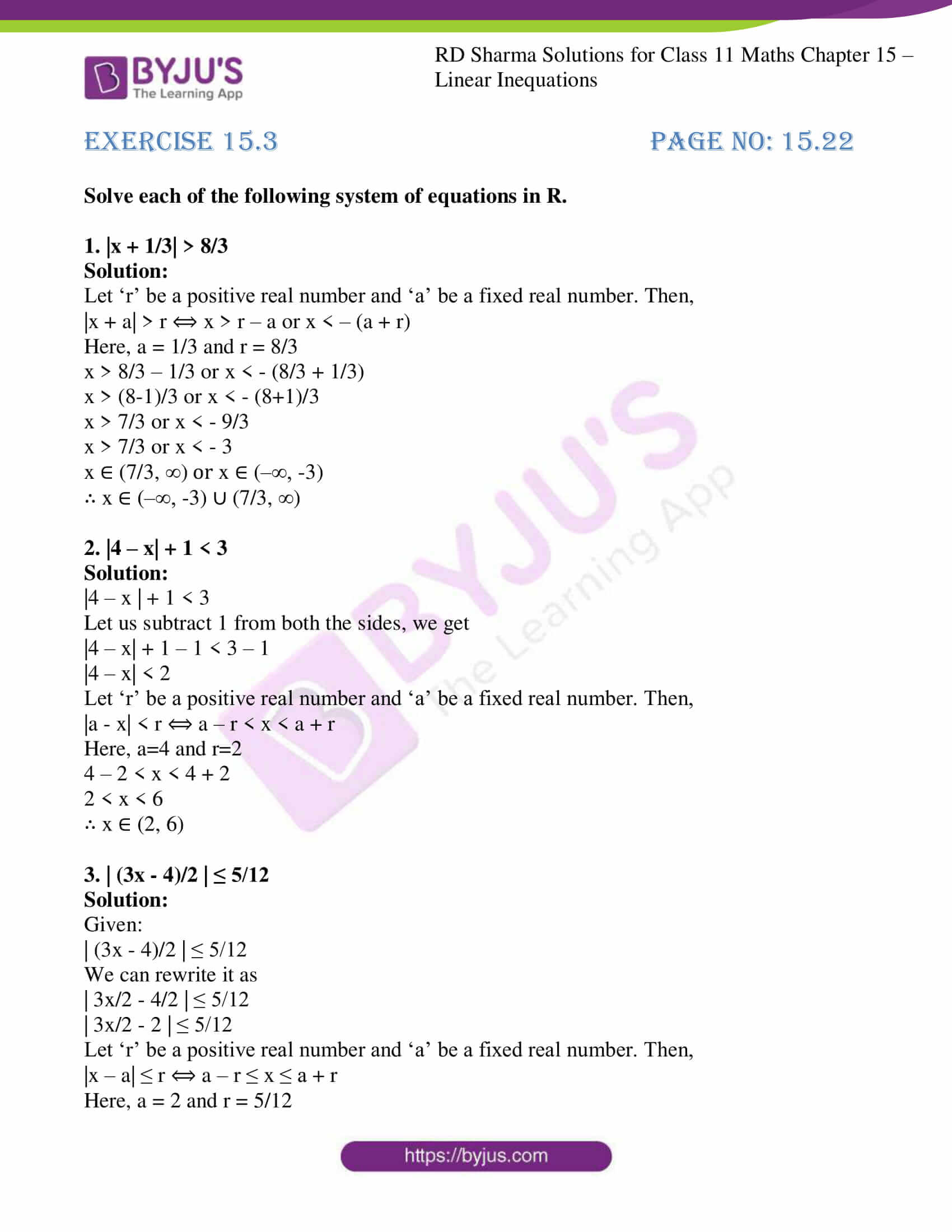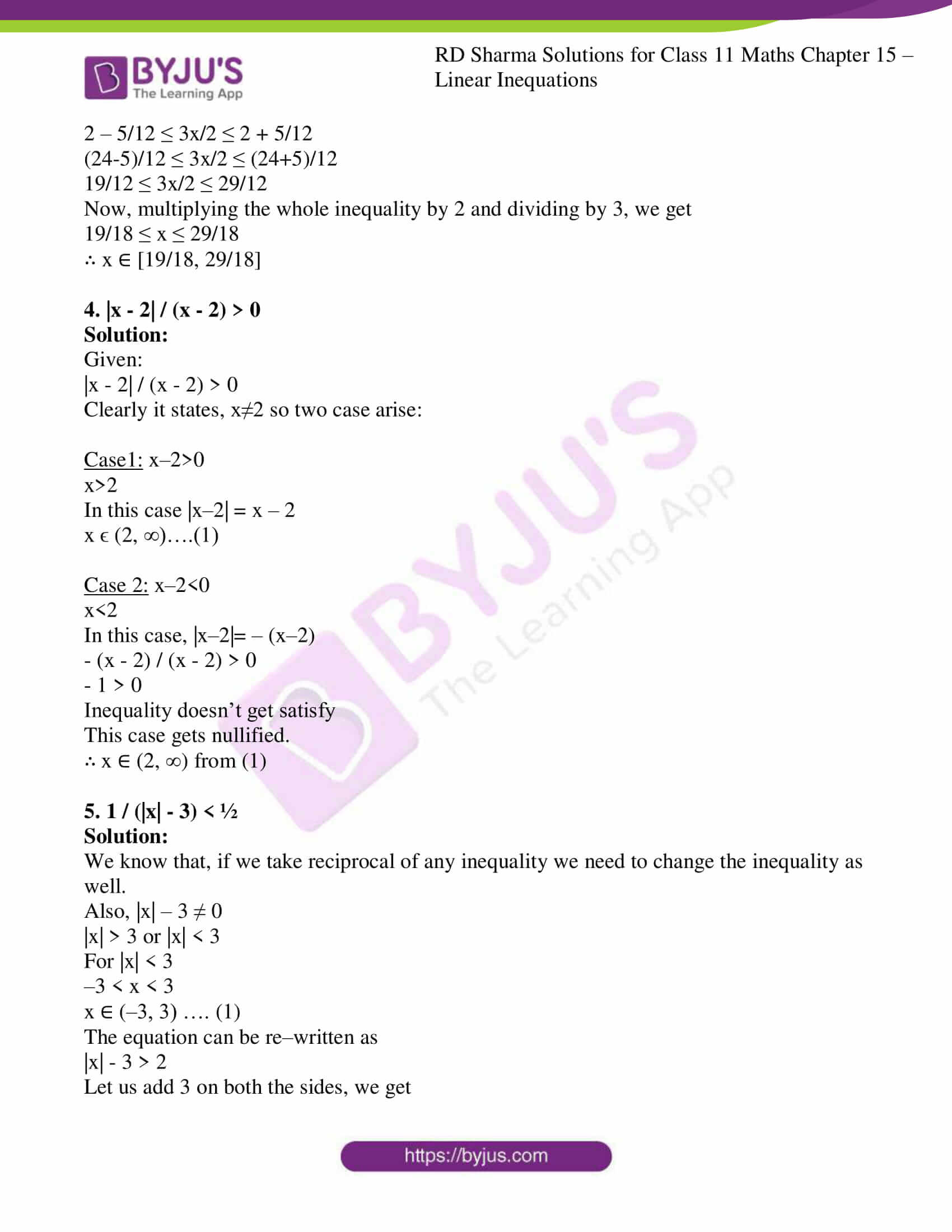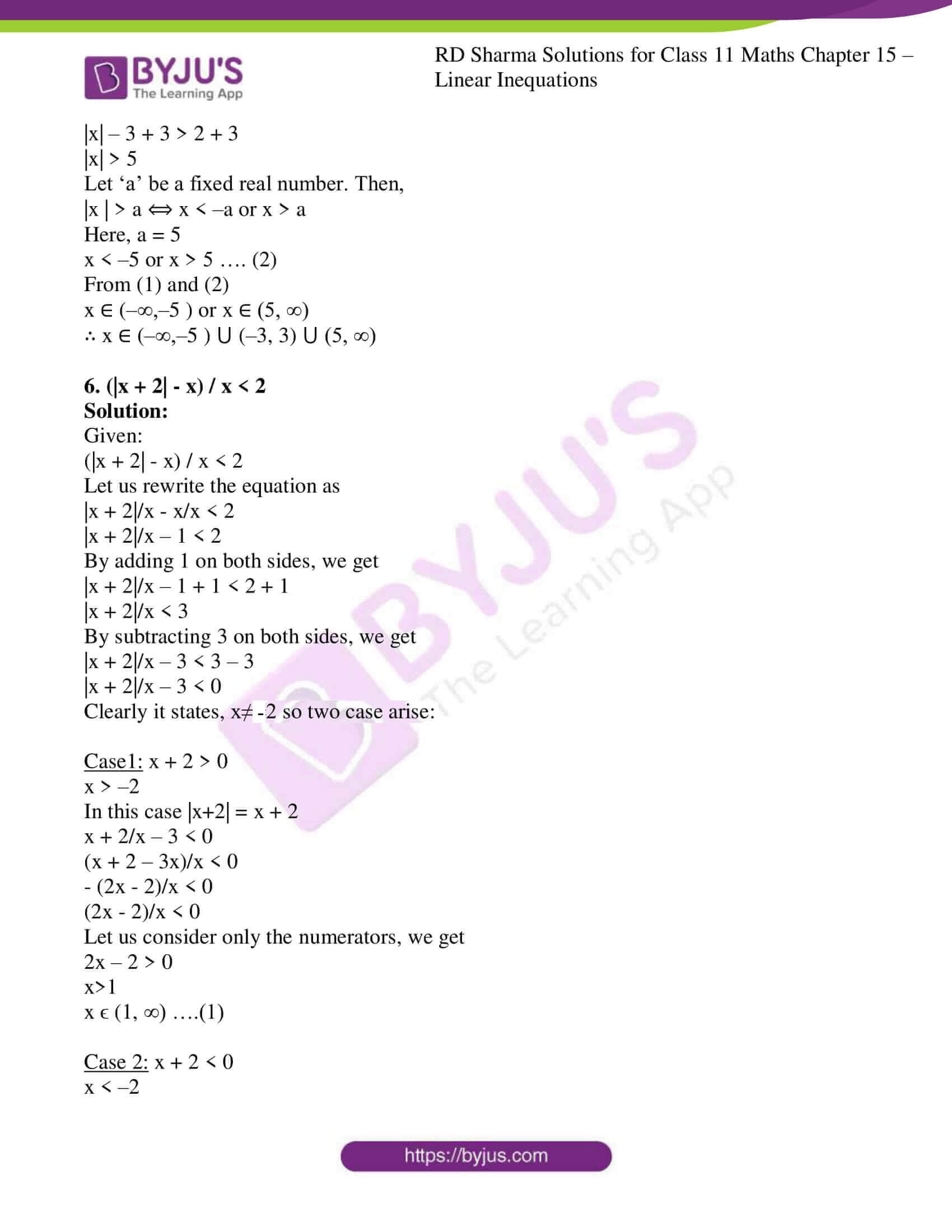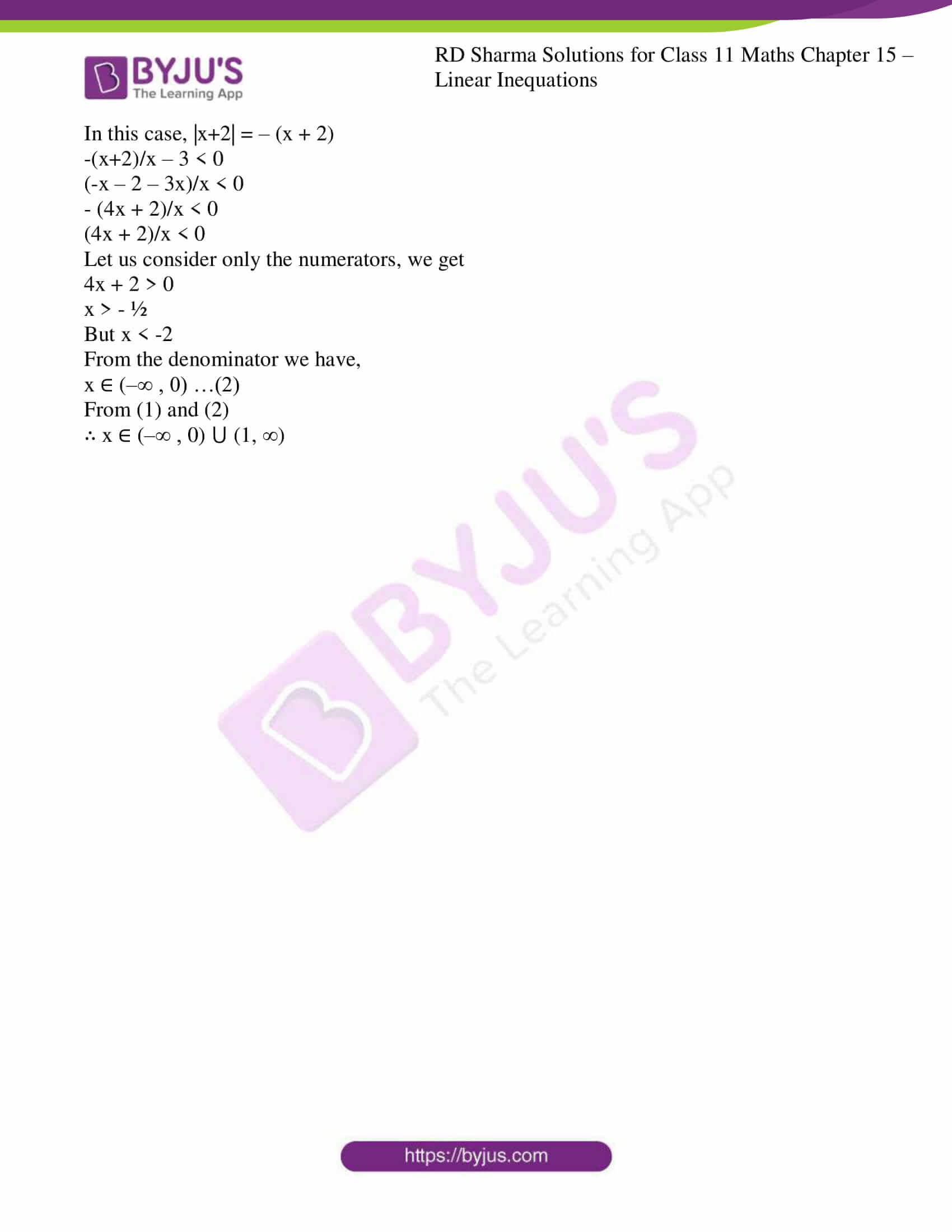# RD Sharma Solutions for Class 11 Chapter 15 - Linear Inequations Exercise 15.3

In this sub-section, we shall discuss some results on inequations involving modulus of the variable. We state and prove these results as theorems.

Students having any difficulties in solving the problems can use RD Sharma Class 11 Solutions as reference material. Our experts have solved the problems in a simple and easily understandable manner, which any student can understand and thereby, score well in their exams. To access RD Sharma Class 11 Maths Solutions students have to download the pdf from the links given below.

## Download the pdf of RD Sharma Solutions for Class 11 Maths Exercise 15.3 Chapter 15 – Linear Inequations### Also, access other exercises of RD Sharma Solutions for Class 11 Maths Chapter 15 – Linear Inequations

Exercise 15.1 Solutions

Exercise 15.2 Solutions

Exercise 15.4 Solutions

Exercise 15.5 Solutions

Exercise 15.6 Solutions

### Access answers to RD Sharma Solutions for Class 11 Maths Exercise 15.3 Chapter 15 – Linear Inequations

Solve each of the following system of equations in R.

1. |x + 1/3| > 8/3

Solution:

Let ‘r’ be a positive real number and ‘a’ be a fixed real number. Then,

|x + a| > r ⟺ x > r – a or x < – (a + r)

Here, a = 1/3 and r = 8/3

x > 8/3 – 1/3 or x < – (8/3 + 1/3)

x > (8-1)/3 or x < – (8+1)/3

x > 7/3 or x < – 9/3

x > 7/3 or x < – 3

x ∈ (7/3, ∞) or x ∈ (–∞, -3)

∴ x ∈ (–∞, -3) ∪ (7/3, ∞)

2. |4 – x| + 1 < 3

Solution:

|4 – x | + 1 < 3

Let us subtract 1 from both the sides, we get

|4 – x| + 1 – 1 < 3 – 1

|4 – x| < 2

Let ‘r’ be a positive real number and ‘a’ be a fixed real number. Then,

|a – x| < r ⟺ a – r < x < a + r

Here, a=4 and r=2

4 – 2 < x < 4 + 2

2 < x < 6

∴ x ∈ (2, 6)

3. | (3x – 4)/2 | ≤ 5/12

Solution:

Given:

| (3x – 4)/2 | ≤ 5/12

We can rewrite it as

| 3x/2 – 4/2 | ≤ 5/12

| 3x/2 – 2 | ≤ 5/12

Let ‘r’ be a positive real number and ‘a’ be a fixed real number. Then,

|x – a| ≤ r ⟺ a – r ≤ x ≤ a + r

Here, a = 2 and r = 5/12

2 – 5/12 ≤ 3x/2 ≤ 2 + 5/12

(24-5)/12 ≤ 3x/2 ≤ (24+5)/12

19/12 ≤ 3x/2 ≤ 29/12

Now, multiplying the whole inequality by 2 and dividing by 3, we get

19/18 ≤ x ≤ 29/18

∴ x ∈ [19/18, 29/18]

4. |x – 2| / (x – 2) > 0

Solution:

Given:

|x – 2| / (x – 2) > 0

Clearly it states, x≠2 so two case arise:

Case1: x–2>0

x>2

In this case |x–2| = x – 2

x ϵ (2, ∞)….(1)

Case 2: x–2<0

x<2

In this case, |x–2|= – (x–2)

– (x – 2) / (x – 2) > 0

– 1 > 0

Inequality doesn’t get satisfy

This case gets nullified.

∴ x ∈ (2, ∞) from (1)

5. 1 / (|x| – 3) < ½

Solution:

We know that, if we take reciprocal of any inequality we need to change the inequality as well.

Also, |x| – 3 ≠ 0

|x| > 3 or |x| < 3

For |x| < 3

–3 < x < 3

x ∈ (–3, 3) …. (1)

The equation can be re–written as

|x| – 3 > 2

Let us add 3 on both the sides, we get

|x| – 3 + 3 > 2 + 3

|x| > 5

Let ‘a’ be a fixed real number. Then,

|x | > a ⟺ x < –a or x > a

Here, a = 5

x < –5 or x > 5 …. (2)

From (1) and (2)

x ∈ (–∞,–5 ) or x ∈ (5, ∞)

∴ x ∈ (–∞,–5 ) ⋃ (–3, 3) ⋃ (5, ∞)

6. (|x + 2| – x) / x < 2

Solution:

Given:

(|x + 2| – x) / x < 2

Let us rewrite the equation as

|x + 2|/x – x/x < 2

|x + 2|/x – 1 < 2

By adding 1 on both sides, we get

|x + 2|/x – 1 + 1 < 2 + 1

|x + 2|/x < 3

By subtracting 3 on both sides, we get

|x + 2|/x – 3 < 3 – 3

|x + 2|/x – 3 < 0

Clearly it states, x ≠ -2 so two case arise:

Case1: x + 2 > 0

x > –2

In this case |x+2| = x + 2

x + 2/x – 3 < 0

(x + 2 – 3x)/x < 0

– (2x – 2)/x < 0

(2x – 2)/x < 0

Let us consider only the numerators, we get

2x – 2 > 0

x>1

x ϵ (1, ∞) ….(1)

Case 2: x + 2 < 0

x < –2

In this case, |x+2| = – (x + 2)

-(x+2)/x – 3 < 0

(-x – 2 – 3x)/x < 0

– (4x + 2)/x < 0

(4x + 2)/x < 0

Let us consider only the numerators, we get

4x + 2 > 0

x > – ½

But x < -2

From the denominator we have,

x ∈ (–∞ , 0) …(2)

From (1) and (2)

∴ x ∈ (–∞ , 0) ⋃ (1, ∞)